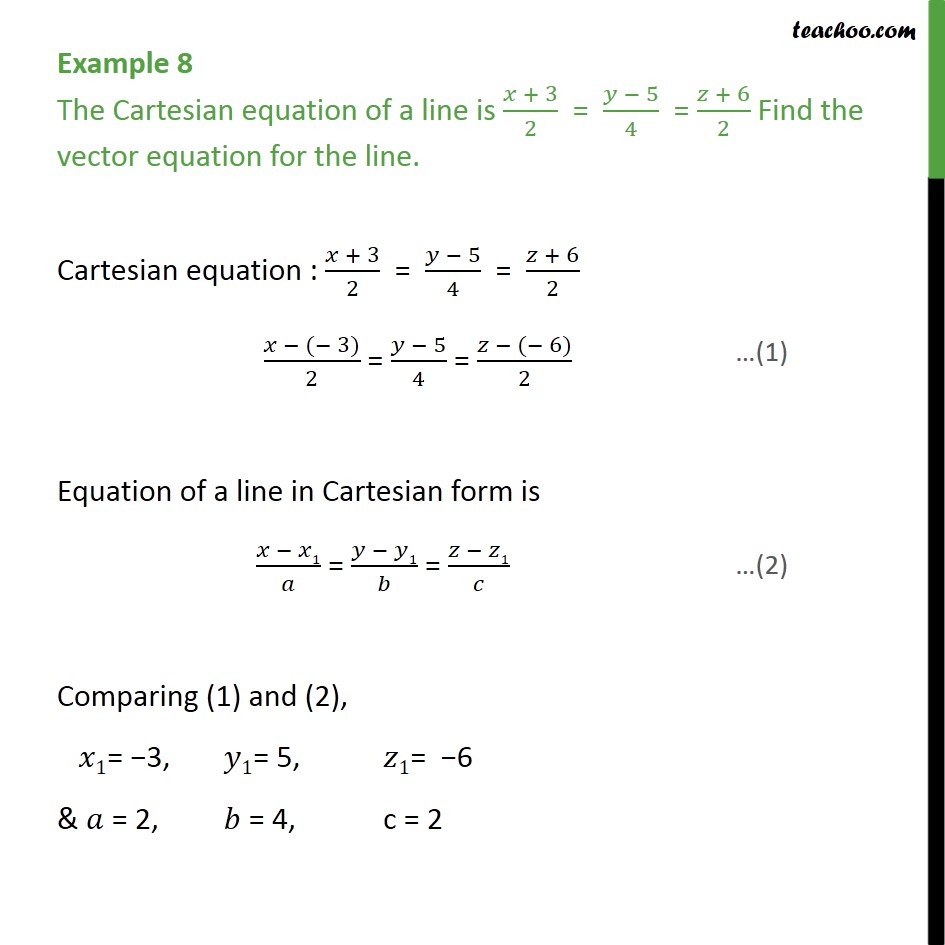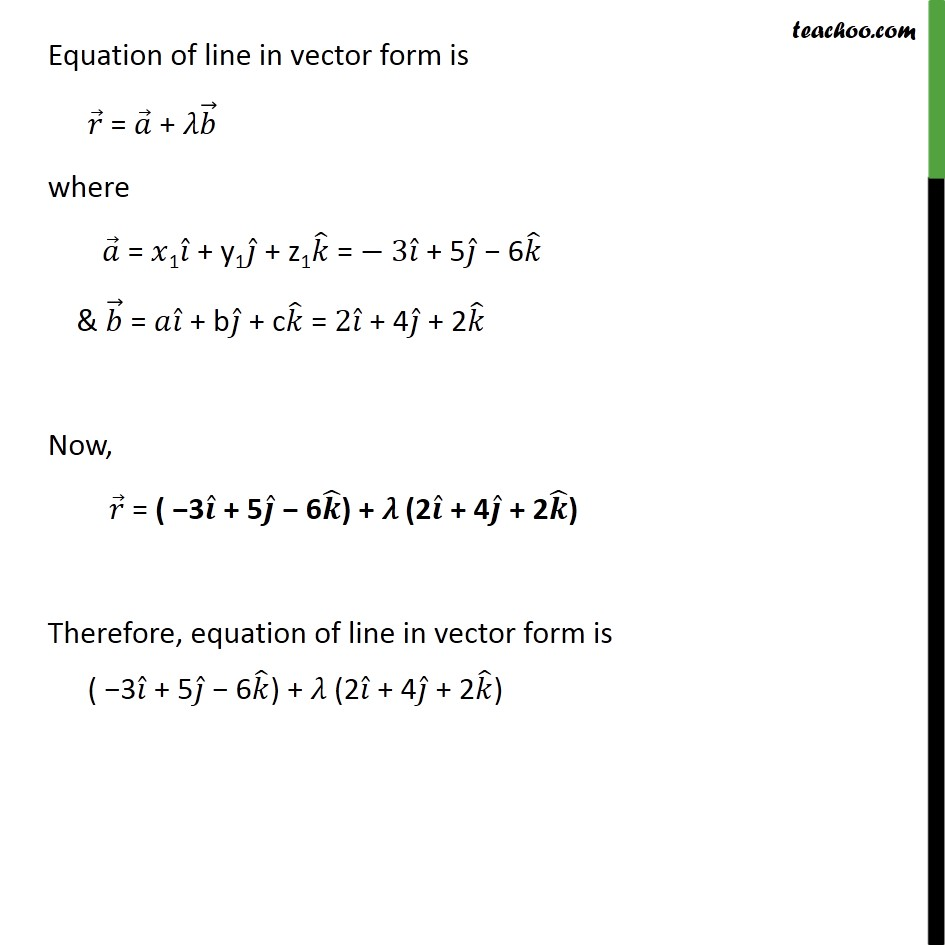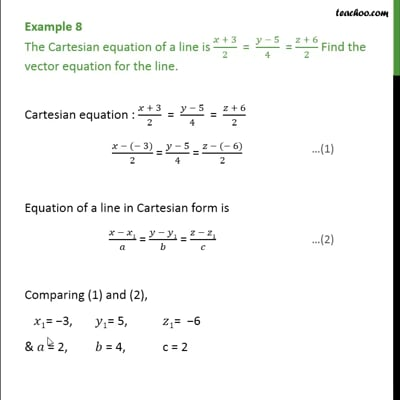Examples

Chapter 11 Class 12 Three Dimensional Geometry
Serial order wiseThis video is only available for Teachoo black users

Solve all your doubts with Teachoo Black (new monthly pack available now!)

### Transcript

Example 8 The Cartesian equation of a line is + 3 2 = 5 4 = + 6 2 Find the vector equation for the line. Cartesian equation : + 3 2 = 5 4 = + 6 2 ( 3) 2 = 5 4 = ( 6) 2 Equation of a line in Cartesian form is 1 = 1 = 1 Comparing (1) and (2), 1= 3, 1= 5, 1= 6 & = 2, = 4, c = 2 Equation of line in vector form is = + where = 1 + y1 + z1 = 3 + 5 6 & = + b + c = 2 + 4 + 2 Now, = ( 3 + 5 6 ) + (2 + 4 + 2 ) Therefore, equation of line in vector form is ( 3 + 5 6 ) + (2 + 4 + 2 )